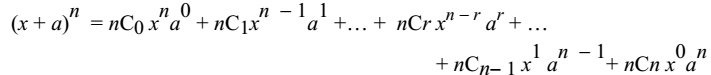# Binomial Theorem

A Binomial is an algebraic expression of two terms which are connected by the operation: + or −.

For example: x+2y, x−y, x3+4y, a+b

Finding squares and cubes of a binomial by actual multiplication is not difficult. But the process of finding the expansion of binomials with higher powers becomes more difficult. There is a general formula which can help in finding the expansion of binomials with higher powers.

Binomial Theorem for Positive Integral IndexGeneral Term

Tr+1 = nCr xn−r ar

### Properties

1. There are (n+1) terms in the expansion of (x+a)n.
2. The degree of x in each term decreases while that of a increases such that the sum of the powers in each term is equal to n.
3. nC0, nC1, nC2, nCrnCn are called binomial coefficients.
4. Since nCr = nCn−r, the coefficients of terms equidistant from the beginning and the end are equal.
5. The binomial coefficients of the various terms of the expansion of (x+a)n for n = 1, 2, 3 form a pattern.

### Pascal's Triangle

The arrangement of the binomial coefficients is known as Pascal’s triangle. The first and last numbers are 1 each. The other numbers are obtained by adding the left and right numbers in the previous row.